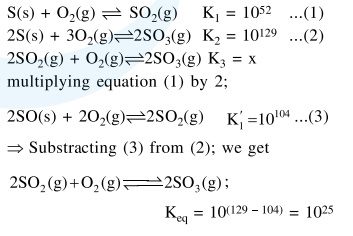Deepak Scored 45->99%ile with Bounce Back Crack Course. You can do it too!

# For the following reactions,

Question:

For the following reactions, equilibrium constants are given :

$\mathrm{S}(\mathrm{s})+\mathrm{O}_{2}(\mathrm{~g}) \rightleftharpoons \mathrm{SO}_{2}(\mathrm{~g}) ; \mathrm{K}_{1}=10^{52}$

$2 \mathrm{~S}(\mathrm{~s})+3 \mathrm{O}_{2}(\mathrm{~g}) \rightleftharpoons 2 \mathrm{SO}_{3}(\mathrm{~g}) ; \mathrm{K}_{2}=10^{129}$

The equilibrium constant for the reaction,

$2 \mathrm{SO}_{2}(\mathrm{~g})+\mathrm{O}_{2}(\mathrm{~g}) \rightleftharpoons 2 \mathrm{SO}_{3}(\mathrm{~g})$ is :

1. $10^{181}$

2. $10^{154}$

3. $10^{25}$

4. $10^{77}$

Correct Option: , 3

Solution: